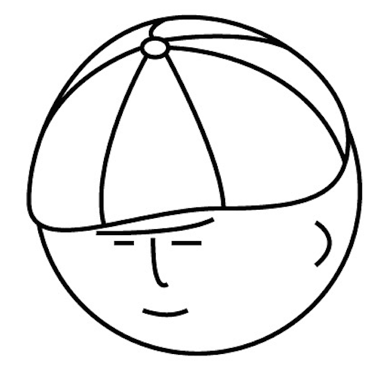# マツシタのお勉強メモ

#### マツシタのお勉強

##### welcome to my engineering blog
###### Hacker Rank# 【グラフ理論とネットワーク理論】Matrix Expression of Graphs and Paths #2

Introduction Here is the article of the last time class. keita-matsushita.hatenablog.com Matrix Expression of Graphs and Paths Graphs can be expressed by matrixes. Below matrixes express above the graph. Incidence matrix (接続行列) Cols re…

# Find an Element from Sorted Matrix

Problem Given an M * N matrix in which each row and each column is sorted in ascending order, write a method to find an element. Solution O(N + M) solution By traversing matrix from upper right to lower left, we can solve this problem in O…

# Range Sum Query 2D - Mutable : Past Google Coding Interview

Problem https://leetcode.com/problems/range-sum-query-2d-mutable/ How to Solve This problem can be solved by using Binary Indexed Tree. Please check below article if you want to know the details of Binary Indexed Tree. keita-matsushita.hat…